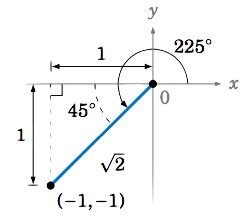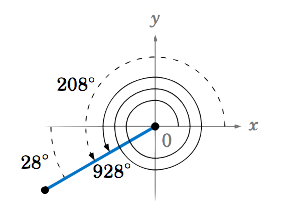$$\newcommand{\id}{\mathrm{id}}$$ $$\newcommand{\Span}{\mathrm{span}}$$ $$\newcommand{\kernel}{\mathrm{null}\,}$$ $$\newcommand{\range}{\mathrm{range}\,}$$ $$\newcommand{\RealPart}{\mathrm{Re}}$$ $$\newcommand{\ImaginaryPart}{\mathrm{Im}}$$ $$\newcommand{\Argument}{\mathrm{Arg}}$$ $$\newcommand{\norm}{\| #1 \|}$$ $$\newcommand{\inner}{\langle #1, #2 \rangle}$$ $$\newcommand{\Span}{\mathrm{span}}$$

1.4: Trigonometric Functions of Any Angle

•• Contributed by Michael Corral
• Professor (Mathematics) at Schoolcraft College

$$\newcommand{\vecs}{\overset { \rightharpoonup} {\mathbf{#1}} }$$

$$\newcommand{\vecd}{\overset{-\!-\!\rightharpoonup}{\vphantom{a}\smash {#1}}}$$Figure 1.4.1 Definition of a general angleFigure 1.4.2 Angle greater than $$360^◦$$Figure 1.4.3 $$x y$$-coordinate plane

Now let $$\theta$$ be any angle. We say that $$\theta$$ is in standard position if its initial side is the positive $$x$$-axis and its vertex is the origin $$(0,0)$$. Pick any point $$(x,y)$$ on the terminal side of $$\theta$$ a distance $$r>0$$ from the origin (see Figure 1.4.3(c)). (Note that $$r = \sqrt{ x^2 + y^2 }$$. Why?) We then define the trigonometric functions of $$\theta$$ as follows:

$\label{1.2} \sin\;\theta ~=~ \dfrac{y}{r} \qquad\qquad \cos\;\theta ~=~ \dfrac{x}{r} \qquad\qquad \tan\;\theta ~=~ \dfrac{y}{x}$

$\label{1.3}\csc\;\theta ~=~ \dfrac{r}{y} \qquad\qquad \sec\;\theta ~=~ \dfrac{r}{x} \qquad\qquad \cot\;\theta ~=~ \dfrac{x}{y}$

As in the acute case, by the use of similar triangles these definitions are well-defined (i.e. they do not depend on which point $$(x,y)$$ we choose on the terminal side of $$\theta$$). Also, notice that $$| \sin\;\theta | \le 1$$ and $$| \cos\;\theta | \le 1$$, since $$| y | \le r$$ and $$| x | \le r$$ in the above definitions.

Notice that in the case of an acute angle these definitions are equivalent to our earlier definitions in terms of right triangles: draw a right triangle with angle $$\theta$$ such that $$x = \text{adjacent side}$$, $$y = \text{opposite side}$$, and $$r = \text{hypotenuse}$$. For example, this would give us $$\sin\;\theta = \frac{y}{r} = \frac{\text{opposite}}{\text{hypotenuse}}$$ and $$\cos\;\theta = \frac{x}{r} = \frac{\text{adjacent}}{\text{hypotenuse}}$$, just as before (see Figure 1.4.4(a)).

Example 1.20

Find the exact values of all six trigonometric functions of $$120^\circ$$.Solution:

We know $$120^\circ = 180^\circ - 60^\circ$$. By Example 1.7 in Section 1.2, we see that we can use the point $$(-1,\sqrt{3})$$ on the terminal side of the angle $$120^\circ$$ in QII, since we saw in that example that a basic right triangle with a $$60^\circ$$ angle has adjacent side of length $$1$$, opposite side of length $$\sqrt{3}$$, and hypotenuse of length $$2$$, as in the figure on the right. Drawing that triangle in QII so that the hypotenuse is on the terminal side of $$120^\circ$$ makes $$r = 2$$, $$x=-1$$, and $$y=\sqrt{3}$$. Hence:

$\nonumber \sin\;120^\circ \;=\; \dfrac{y}{r} \;=\; \dfrac{\sqrt{3}}{2} \qquad \cos\;120^\circ \;=\; \dfrac{x}{r} \;=\; \dfrac{-1}{2} \qquad \tan\;120^\circ \;=\; \dfrac{y}{x} \;=\; \dfrac{\sqrt{3}}{-1} \,=\, -\sqrt{3}$

$\nonumber \csc\;120^\circ \;=\; \dfrac{r}{y} \;=\; \dfrac{2}{\sqrt{3}} \qquad \sec\;120^\circ \;=\; \dfrac{r}{x} \;=\; \dfrac{2}{-1} \;=\; -2 \qquad \cot\;120^\circ \;=\; \dfrac{x}{y} \;=\; \dfrac{-1}{\sqrt{3}}$

Example 1.21

Find the exact values of all six trigonometric functions of $$225^\circ$$.Solution:

We know that $$225^\circ = 180^\circ + 45^\circ$$. By Example 1.6 in Section 1.2, we see that we can use the point $$(-1,-1)$$ on the terminal side of the angle $$225^\circ$$ in QIII, since we saw in that example that a basic right triangle with a $$45^\circ$$ angle has adjacent side of length $$1$$, opposite side of length $$1$$, and hypotenuse of length $$\sqrt{2}$$, as in the figure on the right. Drawing that triangle in QIII so that the hypotenuse is on the terminal side of $$225^\circ$$ makes $$r = \sqrt{2}$$, $$x=-1$$, and $$y=-1$$. Hence:

$\nonumber \sin\;225^\circ \;=\; \dfrac{y}{r} \;=\; \dfrac{-1}{\sqrt{2}} \qquad \cos\;225^\circ \;=\; \dfrac{x}{r} \;=\; \dfrac{-1}{\sqrt{2}} \qquad \tan\;225^\circ \;=\; \dfrac{y}{x} \;=\; \dfrac{-1}{-1} \,=\, 1$

$\nonumber \csc\;225^\circ \;=\; \dfrac{r}{y} \;=\; -\sqrt{2} \qquad \sec\;225^\circ \;=\; \dfrac{r}{x} \;=\; -\sqrt{2} \qquad \cot\;225^\circ \;=\; \dfrac{x}{y} \;=\; \dfrac{-1}{-1} \,=\, 1$

Example 1.22

Find the exact values of all six trigonometric functions of $$330^\circ$$.Solution:

We know that $$330^\circ = 360^\circ - 30^\circ$$. By Example 1.7 in Section 1.2, we see that we can use the point $$(\sqrt{3},-1)$$ on the terminal side of the angle $$225^\circ$$ in QIV, since we saw in that example that a basic right triangle with a $$30^\circ$$ angle has adjacent side of length $$\sqrt{3}$$, opposite side of length $$1$$, and hypotenuse of length $$2$$, as in the figure on the right. Drawing that triangle in QIV so that the hypotenuse is on the terminal side of $$330^\circ$$ makes $$r = 2$$, $$x=\sqrt{3}$$, and $$y=-1$$. Hence:

$\nonumber \sin\;330^\circ \;=\; \dfrac{y}{r} \;=\; \dfrac{-1}{2} \qquad \cos\;330^\circ \;=\; \dfrac{x}{r} \;=\; \dfrac{\sqrt{3}}{2} \qquad \tan\;330^\circ \;=\; \dfrac{y}{x} \;=\; \dfrac{-1}{\sqrt{3}}$

$\nonumber \csc\;330^\circ \;=\; \dfrac{r}{y} \;=\; -2 \qquad \sec\;330^\circ \;=\; \dfrac{r}{x} \;=\; \dfrac{2}{\sqrt{3}} \qquad \cot\;330^\circ \;=\; \dfrac{x}{y} \;=\; -\sqrt{3}$

Solution:

These angles are different from the angles we have considered so far, in that the terminal sides lie along either the $$x$$-axis or the $$y$$-axis. So unlike the previous examples, we do not have any right triangles to draw. However, the values of the trigonometric functions are easy to calculate by picking the simplest points on their terminal sides and then using the definitions in formulas Equation \ref{1.2} and Equation \ref{1.3}.

For instance, for the angle $$0^\circ$$ use the point $$(1,0)$$ on its terminal side (the positive $$x$$-axis), as in Figure 1.4.6. You could think of the line segment from the origin to the point $$(1,0)$$ as sort of a degenerate right triangle whose height is $$0$$ and whose hypotenuse and base have the same length $$1$$. Regardless, in the formulas we would use $$r = 1$$, $$x = 1$$, and $$y = 0$$. Hence:

$\nonumber \sin\;0^\circ \;=\; \dfrac{y}{r} \;=\; \dfrac{0}{1} \;=\; 0 \qquad \cos\;0^\circ \;=\; \dfrac{x}{r} \;=\; \dfrac{1}{1} \;=\; 1 \qquad \tan\;0^\circ \;=\; \dfrac{y}{x} \;=\; \dfrac{0}{1} \;=\; 0$

$\nonumber \csc\;0^\circ \;=\; \dfrac{r}{y} \;=\; \dfrac{1}{0} \;=\; \text{undefined}\qquad \sec\;0^\circ \;=\; \dfrac{r}{x} \;=\; \dfrac{1}{1} \;=\; 1 \qquad \cot\;0^\circ \;=\; \dfrac{x}{y} \;=\; \dfrac{1}{0} \;=\; \text{undefined}$

Note that $$\csc\;0^\circ$$ and $$\cot\;0^\circ$$ are undefined, since division by $$0$$ is not allowed.

Similarly, from Figure 1.4.6 we see that for $$90^\circ$$ the terminal side is the positive $$y$$-axis, so use the point $$(0,1)$$. Again, you could think of the line segment from the origin to $$(0,1)$$ as a degenerate right triangle whose base has length $$0$$ and whose height equals the length of the hypotenuse. We have $$r = 1$$, $$x = 0$$, and $$y = 1$$, and hence:

$\nonumber \sin\;90^\circ \;=\; \dfrac{y}{r} \;=\; \dfrac{1}{1} \;=\; 1 \qquad \cos\;90^\circ \;=\; \dfrac{x}{r} \;=\; \dfrac{0}{1} \;=\; 0 \qquad \tan\;90^\circ \;=\; \dfrac{y}{x} \;=\; \dfrac{1}{0} \;=\; \text{undefined}$

$\nonumber \csc\;90^\circ \;=\; \dfrac{r}{y} \;=\; \dfrac{1}{1} \;=\; 1\qquad \sec\;90^\circ \;=\; \dfrac{r}{x} \;=\; \dfrac{1}{0} \;=\; \text{undefined}\qquad \cot\;90^\circ \;=\; \dfrac{x}{y} \;=\; \dfrac{0}{1} \;=\; 0$

Likewise, for $$180^\circ$$ use the point $$(-1,0)$$ so that $$r = 1$$, $$x = -1$$, and $$y = 0$$. Hence:

$\nonumber \sin\;180^\circ \;=\; \dfrac{y}{r} \;=\; \dfrac{0}{1} \;=\; 0 \qquad \cos\;180^\circ \;=\; \dfrac{x}{r} \;=\; \dfrac{-1}{1} \;=\; -1 \qquad \tan\;180^\circ \;=\; \dfrac{y}{x} \;=\; \dfrac{0}{-1} \;=\; 0$

$\nonumber \csc\;180^\circ \;=\; \dfrac{r}{y} \;=\; \dfrac{1}{0} \;=\; \text{undefined}\quad\;\;\; \sec\;180^\circ \;=\; \dfrac{r}{x} \;=\; \dfrac{1}{-1} \;=\; -1\quad\;\;\; \cot\;180^\circ \;=\; \dfrac{x}{y} \;=\; \dfrac{-1}{0} \;=\; \text{undefined}$

Lastly, for $$270^\circ$$ use the point $$(0,-1)$$ so that $$r = 1$$, $$x = 0$$, and $$y = -1$$. Hence:

$\nonumber \sin\;270^\circ \;=\; \dfrac{y}{r} \;=\; \dfrac{-1}{1} \;=\; -1 \qquad \cos\;270^\circ \;=\; \dfrac{x}{r} \;=\; \dfrac{0}{1} \;=\; 0 \qquad \tan\;270^\circ \;=\; \dfrac{y}{x} \;=\; \dfrac{-1}{0} \;=\; \text{undefined}$

$\nonumber \csc\;270^\circ \;=\; \dfrac{r}{y} \;=\; \dfrac{1}{-1} \;=\; -1\qquad \sec\;270^\circ \;=\; \dfrac{r}{x} \;=\; \dfrac{1}{0} \;=\; \text{undefined}\qquad \cot\;270^\circ \;=\; \dfrac{x}{y} \;=\; \dfrac{0}{-1} \;=\; 0$

The following table summarizes the values of the trigonometric functions of angles between $$0^\circ$$ and $$360^\circ$$ which are integer multiples of $$30^\circ$$ or $$45^\circ$$:

Table 1.3 Table of trigonometric function valuesSince $$360^\circ$$ represents one full revolution, the trigonometric function values repeat every $$360^\circ$$. For example, $$\sin\;360^\circ = \sin\;0^\circ$$, $$\cos\;390^\circ = \cos\;30^\circ$$, $$\tan\;540^\circ = \tan\;180^\circ$$, $$\sin\;(-45^\circ) = \sin\;315^\circ$$, etc. In general, if two angles differ by an integer multiple of $$360^\circ$$ then each trigonometric function will have equal values at both angles. Angles such as these, which have the same initial and terminal sides, are called coterminal.

In Examples 1.20-1.22, we saw how the values of trigonometric functions of an angle $$\theta$$ larger than $$90^\circ$$ were found by using a certain acute angle as part of a right triangle. That acute angle has a special name: if $$\theta$$ is a nonacute angle then we say that the reference angle for $$\theta$$ is the acute angle formed by the terminal side of $$\theta$$ and either the positive or negative $$x$$-axis. So in Example 1.20, we see that $$60^\circ$$ is the reference angle for the nonacute angle $$\theta = 120^\circ$$; in Example 1.21, $$45^\circ$$ is the reference angle for $$\theta = 225^\circ$$; and in Example 1.22, $$30^\circ$$ is the reference angle for $$\theta = 330^\circ$$.

Example 1.24

Let $$\theta = 928^\circ$$.Figure 1.4.7

1. Which angle between $$0^\circ$$ and $$360^\circ$$ has the same terminal side (and hence the same trigonometric function values) as $$\theta\,$$?
2. What is the reference angle for $$\theta\,$$?

Solution

(a) Since $$928^\circ = 2 \times 360^\circ + 208^\circ$$, then $$\theta$$ has the same terminal side as $$208^\circ$$, as in Figure 1.4.7.

(b) $$928^\circ$$ and $$208^\circ$$ have the same terminal side in QIII, so the reference angle for $$\theta = 928^\circ$$ is $$208^\circ - 180^\circ = 28^\circ$$.Figure 1.4.8 $$\cos θ = − \frac{4}{ 5}$$

When $$\theta$$ is in QII, we see from Figure 1.4.8(a) that the point $$(-4,3)$$ is on the terminal side of $$\theta$$, and so we have $$x = -4$$, $$y = 3$$, and $$r = 5$$. Thus, $$\sin\;\theta = \frac{y}{r} = \frac{3}{5}$$ and $$\tan\;\theta = \frac{y}{x} = \frac{3}{-4}$$.

When $$\theta$$ is in QIII, we see from Figure 1.4.8(b) that the point $$(-4,-3)$$ is on the terminal side of $$\theta$$, and so we have $$x = -4$$, $$y = -3$$, and $$r = 5$$. Thus, $$\sin\;\theta = \frac{y}{r} = \frac{-3}{5}$$ and $$\tan\;\theta = \frac{y}{x} = \frac{-3}{-4} = \frac{3}{4}$$.

Thus, either $$\fbox{\(\sin\;\theta = \frac{3}{5}$$ and $$\tan\;\theta = -\frac{3}{4}$$}\) or $$\fbox{\(\sin\;\theta = -\frac{3}{5}$$ and $$\tan\;\theta = \frac{3}{4}$$}\).

Since reciprocals have the same sign, $$\csc\;\theta$$ and $$\sin\;\theta$$ have the same sign, $$\sec\;\theta$$ and $$\cos\;\theta$$ have the same sign, and $$\cot\;\theta$$ and $$\tan\;\theta$$ have the same sign. So it suffices to remember the signs of $$\sin\;\theta$$, $$\cos\;\theta$$, and $$\tan\;\theta$$:

For an angle $$θ$$ in standard position and a point $$(x, y)$$ on its terminal side:

1. $$\sin\;\theta$$ has the same sign as $$y$$
2. $$\cos\;\theta$$ has the same sign as $$x$$
3. $$\tan\;\theta$$ is positive when $$x$$ and $$y$$ have the same sign
4. $$\tan\;\theta$$ is negative when $$x$$ and $$y$$ have opposite signs